NEET  >  Test: Motion in a Plane - 1

# Test: Motion in a Plane - 1 - NEET

Test Description

## 30 Questions MCQ Test Physics Class 11 - Test: Motion in a Plane - 1

Test: Motion in a Plane - 1 for NEET 2023 is part of Physics Class 11 preparation. The Test: Motion in a Plane - 1 questions and answers have been prepared according to the NEET exam syllabus.The Test: Motion in a Plane - 1 MCQs are made for NEET 2023 Exam. Find important definitions, questions, notes, meanings, examples, exercises, MCQs and online tests for Test: Motion in a Plane - 1 below.
Solutions of Test: Motion in a Plane - 1 questions in English are available as part of our Physics Class 11 for NEET & Test: Motion in a Plane - 1 solutions in Hindi for Physics Class 11 course. Download more important topics, notes, lectures and mock test series for NEET Exam by signing up for free. Attempt Test: Motion in a Plane - 1 | 30 questions in 30 minutes | Mock test for NEET preparation | Free important questions MCQ to study Physics Class 11 for NEET Exam | Download free PDF with solutions
 1 Crore+ students have signed up on EduRev. Have you?
Test: Motion in a Plane - 1 - Question 1

### The basic difference between a scalar and vector is one of

Detailed Solution for Test: Motion in a Plane - 1 - Question 1

Scalar quantity gives you an idea about how much of an object there is, but vector quantity gives you an indication of how much of an object there is and that also in which direction. So, the main difference between these two quantities is associated with the direction, i.e. scalars do not have direction but vectors do.

Test: Motion in a Plane - 1 - Question 2

### Which is a constant for a freely falling object?

Detailed Solution for Test: Motion in a Plane - 1 - Question 2

Explanation:An object that falls through a vacuum is subjected to only one external force, the gravitational force, expressed as the weight of the object. An object that is moving only because of the action of gravity is said to be free falling and its motion is described by Newton's second law of motion. With algebra we can solve for the acceleration of a free falling object. The acceleration is constant and equal to the gravitational acceleration g which is 9.8 meters per square second at sea level on the Earth. The weight, size, and shape of the object are not a factor in describing a free fall.

Test: Motion in a Plane - 1 - Question 3

### Which of the following statements not true?

Detailed Solution for Test: Motion in a Plane - 1 - Question 3

Explanation:The net acceleration of a particle in circular motion is along the radius of the circle towards the centre Only in case of uniform circular motion.

Test: Motion in a Plane - 1 - Question 4

When a ball is thrown upwards, as it rises, the vertical component of its velocity

Detailed Solution for Test: Motion in a Plane - 1 - Question 4

Explanation:if a ball is thrown upward at an angle? The ball has a vertical and a horizontal component of velocity. The force of gravity is acting on it and its acceleration is in the downward direction. The vertical component of velocity is therefore changing. As the motion and acceleration is in opposite direction so vertical component of velocity decrease.

Test: Motion in a Plane - 1 - Question 5

Detailed Solution for Test: Motion in a Plane - 1 - Question 5

Explanation:Parallelogram law of vector addition is,If two vectors are considered to be the adjacent sides of a Parallelogram, then the resultant of two vectors is given by the vector which is a diagonal passing through the point of contact of two vectors.

Test: Motion in a Plane - 1 - Question 6

Detailed Solution for Test: Motion in a Plane - 1 - Question 6

Explanation:The sum of two or more vectors is called the resultant. The resultant of two vectors can be found using either the triangle method or the parallelogram method.

Test: Motion in a Plane - 1 - Question 7

The path of a projectile is

Detailed Solution for Test: Motion in a Plane - 1 - Question 7

Explanation:A particle with a vertical and horizontal velocity travelling in a gravitational field will trace out a parabola.

Test: Motion in a Plane - 1 - Question 8

The reason why cyclists bank when taking a sharp turn is

Detailed Solution for Test: Motion in a Plane - 1 - Question 8

Explanation:In order to take a safe turn, the cyclist has to bend a little from his vertical position. In this case, a component of the reaction provides the required centripetal force.If q is angle made by the cyclist with the vertical then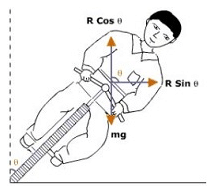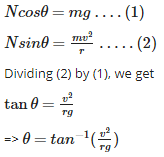In actual practice, the value of q is slightly less because the force of friction also contributes towards the centripetal force.

Test: Motion in a Plane - 1 - Question 9

Magnitude of displacement of a particle is

Detailed Solution for Test: Motion in a Plane - 1 - Question 9

Explanation:The maximum possible value for displacement is the distance travelled, so it cannot be of a greater value than distance (path length).The magnitude of the displacement is always less than or equal to the distance traveled. If two displacements in the same direction are added, then the magnitude of their sum will be equal to the distance traveled.

Test: Motion in a Plane - 1 - Question 10

Detailed Solution for Test: Motion in a Plane - 1 - Question 10

Explanation:Vector addition is the operation of adding two or more vectors together into a vector sum. The so-called parallelogram law gives the rule for vector addition of two or more vectors. For two vectors, the vector sum is obtained by placing them head to tail and drawing the vector from the free tail to the free head.

Test: Motion in a Plane - 1 - Question 11

A motorcycle stunt rider rides off the edge of a cliff. Just at the edge his velocity is horizontal, with magnitude 9.0 m/s. Find the magnitude of the motorcycle’s position vector after 0.50s it leaves the edge of the cliff.

Detailed Solution for Test: Motion in a Plane - 1 - Question 11

Explanation:The motorcycle’s x- and  y-coordinates at t=0.50 s are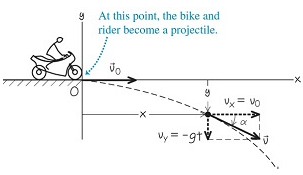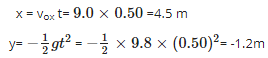The negative value of y shows that the motorcycle is below its starting point.

The motorcycle’s distance from the origin at t=0.50 s is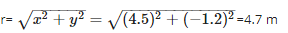Test: Motion in a Plane - 1 - Question 12

Two vectors are equal if

Detailed Solution for Test: Motion in a Plane - 1 - Question 12

Explanation:Equal vectors are vectors that have the same magnitude and the same direction. Equal vectors may start at different positions.

Test: Motion in a Plane - 1 - Question 13

Which of the following physical quantities a vector?

Detailed Solution for Test: Motion in a Plane - 1 - Question 13

Explanation:Angular momentum is a vector quantity (more precisely, a pseudovector) that represents the product of a body's rotational inertia and rotational velocity about a particular axis.

Test: Motion in a Plane - 1 - Question 14

A sports car has a “lateral acceleration” of 0.96g = 9.4 m s−2. This is the maximum centripetal acceleration the car can sustain without skidding out of a curved path. If the car is traveling at a constant 40 m/s on level ground, what is the radius R of the tightest unbanked curve it can negotiate?

Detailed Solution for Test: Motion in a Plane - 1 - Question 14

Explanation:

The car is in uniform circular motion because it’s moving at a constant speed along a curve that is a segment of a circle. Hence we know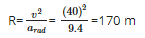This is the minimum turning radius because arad is the maximum centripetal acceleration.

Test: Motion in a Plane - 1 - Question 15

A cyclist moves along a circular path of radius 70m. If he completes one round in 11s, calculate total length of path.

Detailed Solution for Test: Motion in a Plane - 1 - Question 15

Radius of the circular path, r = 70m
Time takes to complete one round, t = 11s
Total length of the path, s = 2πr = 2*22/7*70 = 440m

Test: Motion in a Plane - 1 - Question 16

Which of the following physical quantities a scalar?

Detailed Solution for Test: Motion in a Plane - 1 - Question 16

Work is the energy transferred to an object by force. It is a scalar quantity because it's the product of force and displacement. Work is not the same thing as force, which is a vector quantity, because work is the result of force.

Test: Motion in a Plane - 1 - Question 17

In a harbor, wind is blowing at the speed of 72 km/h and the flag on the mast of a boat anchored in the harbor flutters along the N-E direction. If the boat starts moving at a speed of 51 km/h to the north, what is the direction of the flag on the mast of the boat?

Detailed Solution for Test: Motion in a Plane - 1 - Question 17

Explanation:The flag is fluttering in the north-east direction. It shows that the wind is blowing toward the north-east direction. When the ship begins sailing toward the north, the flag will move along the direction of the relative velocity (vwb) of the wind with respect to the boat.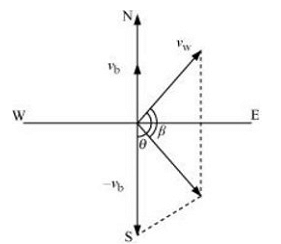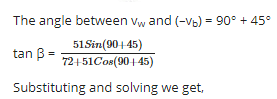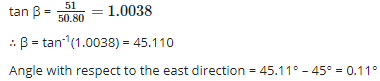Hence, the flag will flutter approximately due east.

Test: Motion in a Plane - 1 - Question 18

Detailed Solution for Test: Motion in a Plane - 1 - Question 18

Explanation:Vector addition is commutative, just like addition of real numbers. If you start from a point say P,  you end up at the same spot no matter which displacement (a or b) you take first. The head-to-tail rule yields vector c for both a + b and b + a.

Test: Motion in a Plane - 1 - Question 19

Which of the following algebraic operations on vectors not permissible?

Detailed Solution for Test: Motion in a Plane - 1 - Question 19

Explanation:Although vectors and scalars represent different types of physical quantities, it is sometimes necessary for them to interact. While adding a scalar to a vector is impossible because of their different dimensions in space, it is possible to multiply a vector by a scalar.

Test: Motion in a Plane - 1 - Question 20

A man can swim with a speed of 4.0 km/h in still water. How long does he take to cross a river 1.0 km wide if the river flows steadily at 3.0 km/h and he makes his strokes normal to the river current? How far down the river does he go when he reaches the other bank?

Detailed Solution for Test: Motion in a Plane - 1 - Question 20

Explanation:

Speed of the man, vm = 4 km/h
Width of the river = 1 km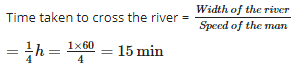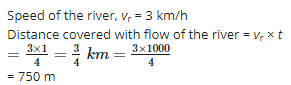Test: Motion in a Plane - 1 - Question 21

The addition of vectors and the multiplication of a vector by a scalar together gives rise to

Detailed Solution for Test: Motion in a Plane - 1 - Question 21

Explanation:

If a vector is multiplied by a scalar as in  pA ,  then the magnitude of the resulting vector is equal to the product of p and the magnitude of  A, and its direction is the same as  A if p is positive and opposite to  A if p is negative.

Distributive law for scalar multiplication:

p(A +B )=pA +pB

Test: Motion in a Plane - 1 - Question 22

Which of the following statements false?

Detailed Solution for Test: Motion in a Plane - 1 - Question 22

Explanation:A displacement is vector that is the shortest path length from the initial to the final position of the body. It is not always eqaul to path length. It can be zero and -ve also.

Test: Motion in a Plane - 1 - Question 23

Rain is falling vertically with a speed of 30 m s−1. A woman rides a bicycle with a speed of 10 m s−1 in the north to south direction. What is the direction in which she should hold her umbrella?

Detailed Solution for Test: Motion in a Plane - 1 - Question 23

The described situation is shown in the given figure.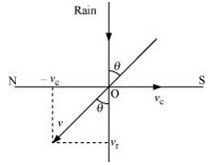Here,

vc = Velocity of the cyclist
vr = Velocity of falling rain

In order to protect herself from the rain, the woman must hold her umbrella in the direction of the relative velocity (v) of the rain with respect to the woman.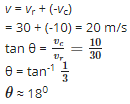Hence, the woman must hold the umbrella toward the south, at an angle of nearly 18° with the vertical.

Test: Motion in a Plane - 1 - Question 24

Null vector or a zero vector has a magnitude

Detailed Solution for Test: Motion in a Plane - 1 - Question 24

Explanation:A null vector is a vector having magnitude equal to zero.It is represented by 0.A null vector has no direction or it may have any direction.Generally a null vector is either equal to resultant of two equal vectors acting in opposite directions or multiple vectors in different directions.

0 =A +(−A )

Test: Motion in a Plane - 1 - Question 25

A passenger arriving in a new town wishes to go from the station to a hotel located 10 km away on a straight road from the station. A dishonest cabman takes him along a circuitous path 23 km long and reaches the hotel in 28 min. What is (a) the average speed of the taxi, (b) the magnitude of average velocity?

Detailed Solution for Test: Motion in a Plane - 1 - Question 25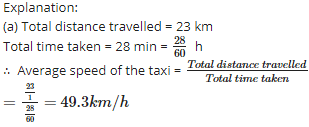(b) Distance between the hostel and the station = 10 km = Displacement of the car

∴ Average velocity =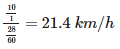Test: Motion in a Plane - 1 - Question 26

A projectile is fired a velocity of 150 meters per second at an angle of 30 degrees with the horizontal. What is the magnitude of the vertical component of the velocity at the time the projectile is fired?

Detailed Solution for Test: Motion in a Plane - 1 - Question 26

Explanation:

Initial velocity u = 150 m/s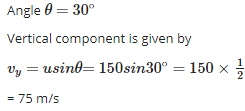Test: Motion in a Plane - 1 - Question 27

Which of the following physical quantities a vector?

Detailed Solution for Test: Motion in a Plane - 1 - Question 27

Explanation:Acceleration is a vector quantity because it has both magnitude and direction.When an object has a positive acceleration, the acceleration occurs in the same direction as the movement of the object.When an object has a negative acceleration (it's slowing down), the acceleration occurs in the opposite direction as the movement of the object.

Test: Motion in a Plane - 1 - Question 28

We can define the difference of two vectors A and B as the

Detailed Solution for Test: Motion in a Plane - 1 - Question 28

Explanation:

Vector subtraction is defined in the following way.

• The difference of two vectors, A - B , is a vector C that is, C = A - B
• The addition of two vector such that C = A + (-B). B has been taken in opposite direction.

Thus vector subtraction can be represented as a vector addition.

Test: Motion in a Plane - 1 - Question 29

Three girls skating on a circular ice ground of radius 200 m start from a point P on the edge of the ground and reach a point Q diametrically opposite to P following different paths as shown in Figure. What is the magnitude of the displacement vector for each ? For which girl is this equal to the actual length of path skate ?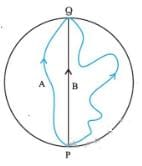Detailed Solution for Test: Motion in a Plane - 1 - Question 29

Explanation:

(i) PQ = diameter = displacement for each girl = 2r = 2 x 200 = 400 m

Since, displacement vector does not depend upon the actual path length and it is the shortest distance between initial and final position, so in the case of each girl the displacement is 400 m.

(ii)This is equal to the actual length of the path skated by girl B.

Test: Motion in a Plane - 1 - Question 30

Which of the following physical quantities a scalar?

Detailed Solution for Test: Motion in a Plane - 1 - Question 30

Explanation:Electric current is a scalar quantity.It represents the direction of flow of positive charge but it is treated as a scalar quantity because current follows the laws of scalar addition and not the laws of vector addition,because the angle between the wires carrying current does not effect the total current in the circuit.

## Physics Class 11

130 videos|483 docs|210 tests
Information about Test: Motion in a Plane - 1 Page
In this test you can find the Exam questions for Test: Motion in a Plane - 1 solved & explained in the simplest way possible. Besides giving Questions and answers for Test: Motion in a Plane - 1, EduRev gives you an ample number of Online tests for practice

## Physics Class 11

130 videos|483 docs|210 tests

### How to Prepare for NEET

Read our guide to prepare for NEET which is created by Toppers & the best Teachers(Scan QR code)